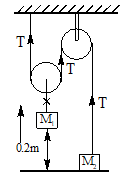In the figure  M1=4M2The pulleys are smooth and light. At time t = 0, the system is at rest. If            the system is released, the acceleration of mass M1 is

# In the figure  M1=4M2The pulleys are smooth and light. At time t = 0, the system is at rest. If            the system is released, the acceleration of mass M1 is1. A

g/2

2. B

g/8

3. C

g/4

4. D

g

Register to Get Free Mock Test and Study Material

+91

Verify OTP Code (required)